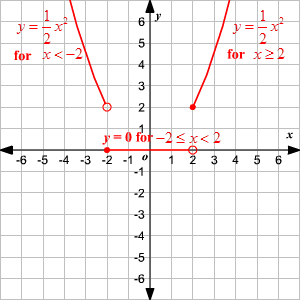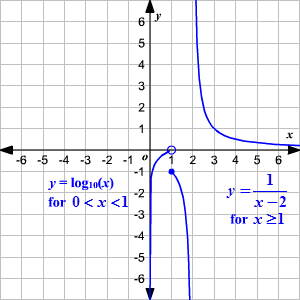# Piecewise-Defined Function

A piecewise-defined function is one which is defined not by a single equation, but by two or more.  Each equation is valid for some interval .

Example 1:

Consider the function defined as follows.The function in this example is piecewise-linear, because each of the three parts of the graph is a line.

Piecewise-defined functions can also have discontinuities ("jumps"). The function in the example below has discontinuities at $x=-2$ and $x=2$ .

Example 2:

Graph the function defined as shown.

Note that we use small white circles in the graph to indicate that the endpoint of a curve is not included in the graph, and solid dots to indicate endpoints that are included.Example 3:

Graph the function defined below.Negative values of $x$ and $0$ are not included in the domain because the first function, $\mathrm{log}x$ , is undefined for those values.  The value $x=2$ is not included in the domain because the second function is not defined for that value (it has a vertical asymptote there). Therefore the domain of this function is $\left\{x\text{\hspace{0.17em}}|\text{\hspace{0.17em}}02\right\}$ . This can be represented using interval notation as $\left(0,2\right)\cup \left(2,\infty \right)$ .